# 考虑嵌入移动孔洞的多相材料布局优化1)

* 大连理工大学 工业装备结构分析国家重点实验室，大连 116023
† 合肥工业大学 土木与水利工程学院，合肥 230009
** 华北电力大学 新能源电力系统国家重点实验室， 北京 102206

# MULTIPHASE MATERIAL LAYOUT OPTIMIZATION CONSIDERING EMBEDDING MOVABLE HOLES1)

Wang Xuan*, Hu Ping*2), Long Kai**

* State Key Laboratory of Structural Analysis for Industrial Equipment， Dalian University of Technology， Dalian 116023, China
† College of Civil Engineering， Hefei University of Technology， Hefei 230009, China
** State Key Laboratory for Alternate Electrical Power System with Renewable Energy Sources， North China Electric Power University，Beijing 102206， China

Abstract

In structural engineering design, it is often necessary to embed one or more fixed-shaped holes to meet certain functional or manufacturing design requirements. To effectively solve the multi-phase material layout optimization problem of continuum structure with embedded movable holes, it is usually necessary to simultaneously optimize the position and orientation of these embedded holes and the topology configuration of the multi-phase material structure to improve the overall performance of the structure. To this end, parameterized level set functions are used to describe the geometry of the embedded holes. The material densities defining the structural topology of multiphase materials, and the geometric parameters used to describe the position and orientation of the embedded holes, are considered as design variables of the optimization problem considered here. To avoid the cumbersome of re-meshing the grids caused by the movement of holes and improve the efficiency of computation, the embedded holes are mapped into a density field on a fixed grid using a smoothed Heaviside function. Meanwhile, a SIMP-like material interpolation invoked at the finite element level is introduced for material parameterization of the optimization problem, and then the simultaneous optimization of the topology configuration of the multi-phase material structure and the position and orientation of the embedded hole can be realized. The material interpolation scheme supports full analytical sensitivity analysis, which allows the current optimization problem to be solved using gradient-based optimization algorithms. Numerical examples illustrate that the proposed method can effectively deal with the layout optimization problem of multiphase material embedded with multiple embedded holes.

Keywords： layout optimization ; movable holes ; multi-material ; SIMP method

0

Wang Xuan, Hu Ping, Long Kai. MULTIPHASE MATERIAL LAYOUT OPTIMIZATION CONSIDERING EMBEDDING MOVABLE HOLES1)[J]. Chinese Journal of Theoretical and Applied Mechanics, 2019, 51(3): 852-862 https://doi.org/10.6052/0459-1879-18-327

topology optimization

## 引 言

Clausen等提出一种有效处理具有灵活空隙区域的拓扑优化方法. 与在设计领域内严格指定空隙区域的被动单元方法不同的是,所建议的方法允许这些区域被灵活地重新定形和重新定位,其中使用惯性矩来控制孔洞的变形程度,使用孔洞区域的质心控制平移运动的自由度. 与文献中的方法的主要区别在于空白定义,允许孔洞空间的完全灵活性. 空隙区域不是使用水平集函数描述,而是通过第二个离散设计变量场引入. 尽管该方法可应用于带有可移动孔洞的布局优化问题,但是该方法在处理带有多个移动孔洞的问题中还存在困难,考虑嵌入$n$个移动孔洞则需要引入$n$个离散的设计变量场,而且还缺少有效避免多个移动孔洞之间的重叠的策略. 另外,文献[17,18]均只涉及单相均质材料的优化问题. 在实际工程应用中,结构通常由多种非均匀材料组成,以满足不同的设计要求,如文献指出多相材料组合有利于减轻结构重量.

## 1 考虑嵌入孔洞的拓扑优化列式

### 1.1 嵌入孔洞的拓扑描述

$$\left.\begin{array}{ll} {\phi \left( x \right) > 0} \,, & {\rm if} \ {\pmb x} \in \left( {D/ \varOmega } \right) {\phi \left( x \right) = 0} \,, & {\rm if} \ {\pmb x} \in \left( {\partial \varOmega \cap D} \right) {\phi \left( x \right) < 0} \,, & {\rm if} \ {\pmb x} \in \left( {\varOmega / \partial \varOmega } \right) \end{array} \!\! \right \} (1)$$

$$\phi \left( {{\pmb x}, x_0 ,y_0 ,\theta } \right) = \phi \left( {\bar {x},\bar {y}} \right) (2)$$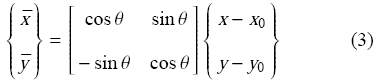$$\phi _1 = \left( {\dfrac{\bar {x}}{a}} \right)^4 + \left( {\dfrac{\bar{y}}{b}} \right)^4-1 (4)$$

$$\eta _e = \prod\limits_{k = 1}^{N_k } \dfrac{1}{1 + \exp \left( { - \beta \phi _k } \right)} (5)$$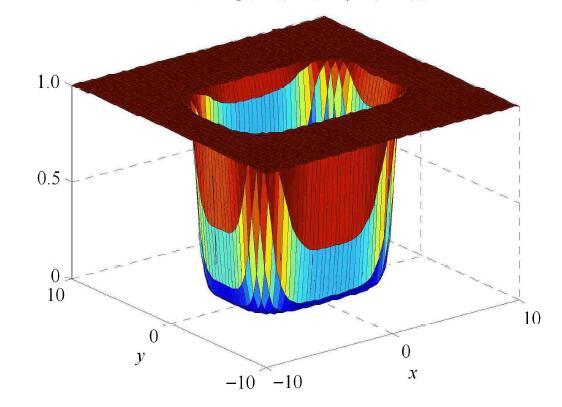Fig. 1   Density of element $e$ mapped from a single moving hole

### 1.2 考虑嵌入孔洞的材料插值格式

$$E_e = \rho _e^p \eta _e^q E_0 (6)$$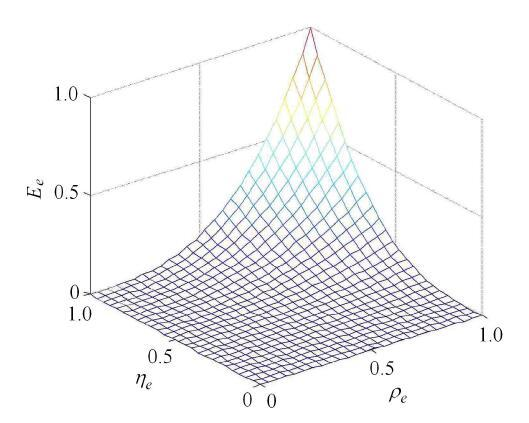Fig. 2   Schematic illustration of SIMP-liked interpolation scheme

$E_e \left( {\rho _1^e ,\rho _2^e } \right) = \left( {\rho _1^e } \right)^{p_1 }\left[{E_1 + \left( {\rho _2^e } \right)^{p_2 }\left( {E_2 - E_1 } \right)} \right]\eta _e^q (7)$

### 1.3 考虑嵌入孔洞的拓扑优化模型

$\left. \begin{matrix} {\rm find}: {\pmb\rho}_1 = \left\{ {\rho _1^1 , \rho _1^2 , \cdots ,\rho _1^{N_e } } \right\} \\ {\pmb\rho}_2 = \left\{ {\rho _2^1 ,\rho _2^2 , \cdots ,\rho _2^{N_e } } \right\} \\ {\pmb s} = \left\{ {s_1 ,s_2 , \cdots ,s_{N_m } } \right\} \\ {\rm min}: \ J = \sum_{e = 1}^{N_e } E_e {\pmb u}_e^T {\pmb k}_0 {\pmb u}_e \\ {\rm s.t.} {\pmb K}{\pmb U} = {\pmb F} \\ g_1 = \sum _{e = 1}^{N_e } \rho _1^e V_e - f_1 V_0 \leqslant 0 \\ g_2 = \sum_{e = 1}^{N_e } \rho _1^e \rho _2^e V_e - f_2 V_0 \leqslant 0 \\ g_3 = \sum_{e = 1}^{N_e } \eta _e V_e - \left( {V_0 - \sum_{k = 1}^{N_m } V_k } \right) \leqslant 0 \\ 0 ＜ \rho _{\min } \leqslant \rho _1^e \leqslant 1 \\ 0 ＜ \rho _{\min } \leqslant \rho _2^e \leqslant 1 \\ s_{\min } \leqslant s \leqslant s_{\max } \end{matrix} \right\} (8)$

## 2 灵敏度分析

### 2.1 目标函数对单元密度变量的灵敏度

$$\dfrac{\partial J}{\partial \rho _i^e } = - \dfrac{\partial E_e }{\partial \rho _i^e }{\pmb u}_e^{\rm T} {\pmb k}_0 {\pmb u}_e \,, \ \ \ i = 1,2 (9)$$

$$\left.\begin{matrix} \dfrac{\partial E_e }{\partial \rho _1^e } = p_1 \left( {\rho _1^e } \right)^{p_1 - 1}\left[ {E_1 + \left( {\rho _2^e } \right)^{p_2 }\left( {E_2 - E_1 } \right)} \right] \eta _e^q \\ \dfrac{\partial E_e }{\partial \rho _2^e } = p_2 \left( {\rho _1^e } \right)^{p_1 }\left( {\rho _2^e } \right)^{p_2 - 1}\left( {E_2 - E_1 } \right) \eta _e^q \\\end{matrix} \right\} (10)$$

### 2.2 目标函数对几何变量的灵敏度

$$\dfrac{\partial J}{\partial z_k } = \sum_{e = 1}^{N_e } \dfrac{\partial J}{\partial \eta _e }\dfrac{\partial \eta _e }{\partial \phi _k }\dfrac{\partial \phi _k }{\partial z_k } (11)$$

$$\dfrac{\partial J}{\partial \eta _e } = - \dfrac{\partial E_e }{\partial\eta _e }{\pmb u}_e^{\rm T} {\pmb k}_0{\pmb u}_e (12)$$

$$\dfrac{\partial E_e }{\partial \eta _e } = q\left( {\rho _1^e } \right)^{p_1 }\left[ {E_1 + \left( {\rho _2^e} \right)^{p_2 }\left( {E_2 - E_1 } \right)} \right]\eta _e^{q - 1} (13)$$

$$\dfrac{\partial \eta _e }{\partial \phi _k } = \eta _e \dfrac{\beta \exp\left( { - \beta \phi _k } \right)}{1 + \exp \left( { - \beta \phi _k} \right)} (14)$$

$$\dfrac{\partial \phi _k }{\partial z_k } = 4\left( {\dfrac{\bar{x}}{a}} \right)^3\left( {\dfrac{ - \cos \theta }{a}} \right) + 4\left({\dfrac{\bar {y}}{b}} \right)^3\left( {\dfrac{\sin \theta }{b}} \right) (15)$$

$$\dfrac{\partial \phi _k }{\partial z_k } = 4\left( {\dfrac{\bar{x}}{a}} \right)^3\left( {\dfrac{ - \sin \theta }{a}} \right) + 4\left({\dfrac{\bar {y}}{b}} \right)^3\left( {\dfrac{ - \cos \theta }{b}} \right) (16)$$

$\dfrac{\partial \phi _k }{\partial z_k } = 4\left( {\dfrac{\bar {x}}{a}} \right)^3\dfrac{ -\sin \theta \cdot \left( {x - x_0 } \right) + \cos \theta \cdot \left( {y - y_0 } \right)}{a} +$

$\qquad 4\left( {\dfrac{\bar {y}}{b}} \right)^3\dfrac{ - \cos \theta \cdot \left( {x - x_0 } \right) -\sin \theta \cdot \left( {y - y_0 } \right)}{b} (17)$

## 3 数值处理

$$\dfrac{\partial J}{\partial \rho _i } = \dfrac{\sum_{j = 1}^{N_i }{w\left( {{\pmb x}_j } \right)\rho _j \dfrac{\partial J}{\partial \rho _j }} }{\rho_i \sum_{j = 1}^{N_i } {w\left( {{\pmb x}_j } \right)} } (18)$$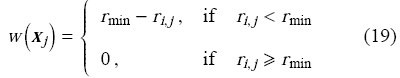$$\dfrac{\left\| {{\pmb\rho}_k - {\pmb\rho}_{k - 1} } \right\|_2 }{\left\| {{\pmb\rho}_k } \right\|_2 } \leqslant \varepsilon (20)$$

## 4 数值算例与讨论

### 4.1 悬臂梁：非重叠约束有效性验证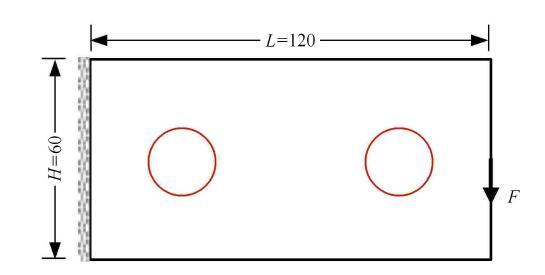Fig. 3   Design domain and boundary condition of cantilever beam problem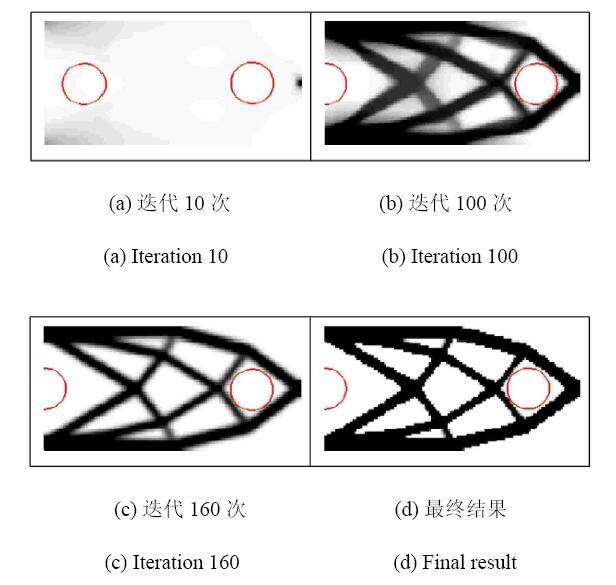Fig. 4   Optimization results of cantilever beam without considering the non-overlap constraint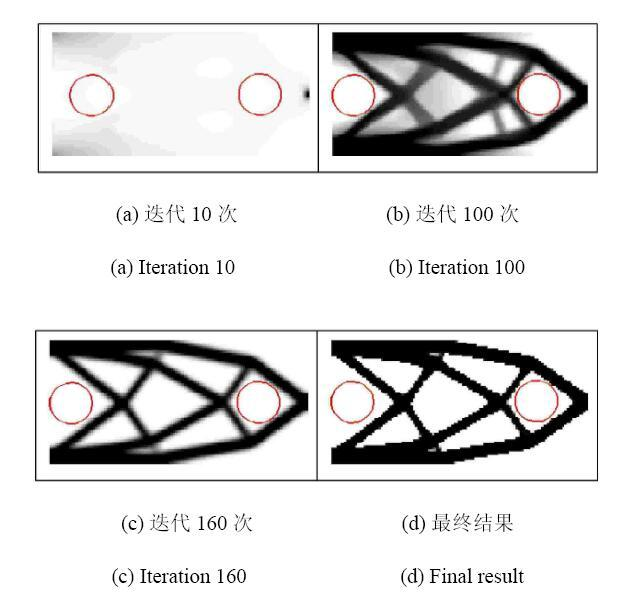Fig. 5   Optimization results of cantilever beam with considering the non-overlap constraint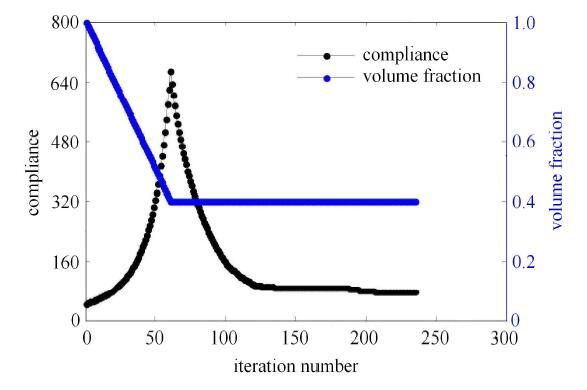Fig. 6   Convergence histories of the objective function and volume fraction

### 4.2 MBB梁：讨论孔洞位置、大小、方向的影响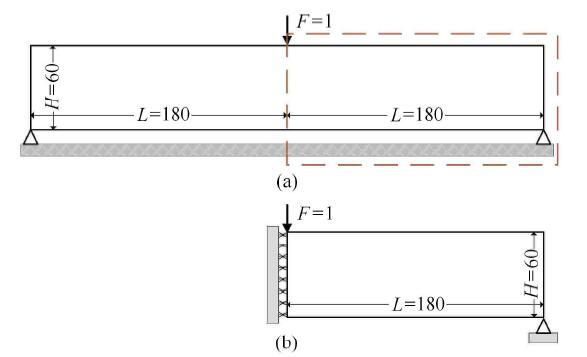Fig. 7   Design domain and boundary condition of MBB beam problem

Table 1   Optimization results for different initial layouts of the embedded holes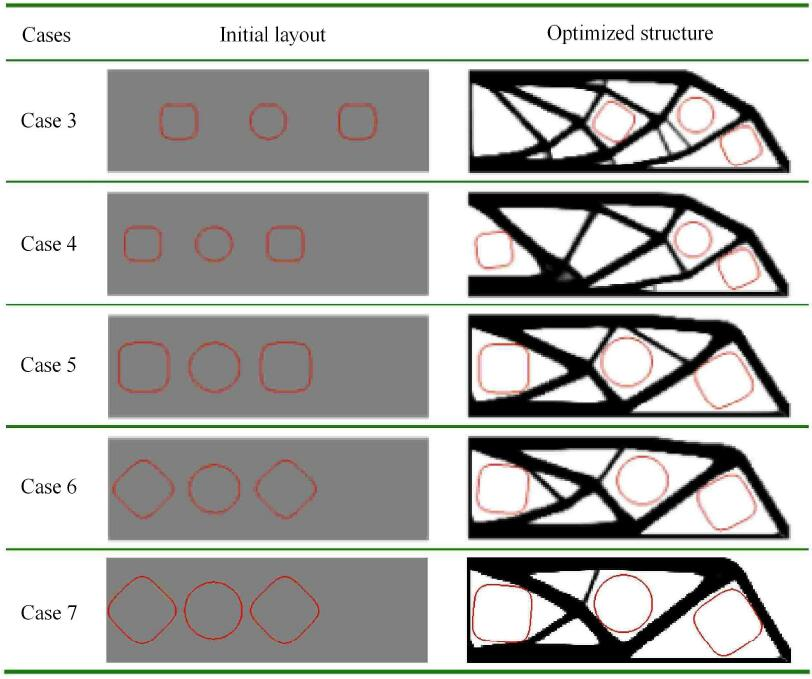Table 2   Optimization results for different initial layouts of the embedded holes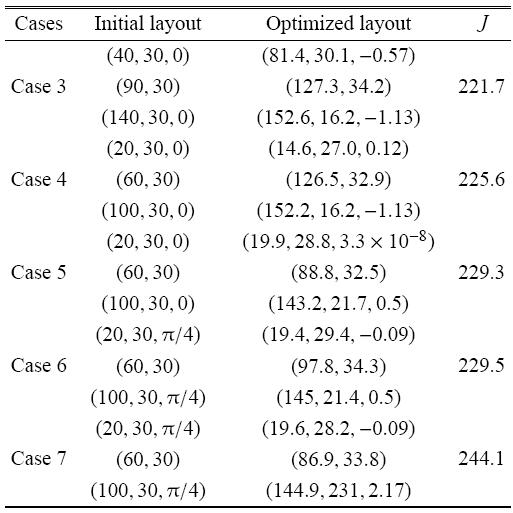### 4.3 L型梁：多相材料布局优化问题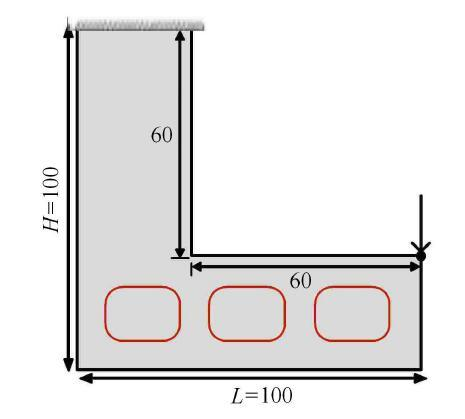Fig. 8   Design domain and boundary condition of L-shaped beam problem

3个孔洞中心的初始位置分别为(20,20),(50,20)和(80,20). 结构顶部边界固支,右边界顶部受单位集中载荷$F = 1$作用.对于两种固体材料,体积分数约束均设置为$20%$,即整个设计域的$60%$为空洞.设计域由6,400个四边形平面应力单元来离散.为了简单起见,我们采用第3节介绍的第一阶段收敛策略,即在整个多相材料设计过程中始终使用由式(18)定义的过滤技术.两个密度变量$\rho _1$和$\rho _2$的容许收敛误差均设置为$0.1%$.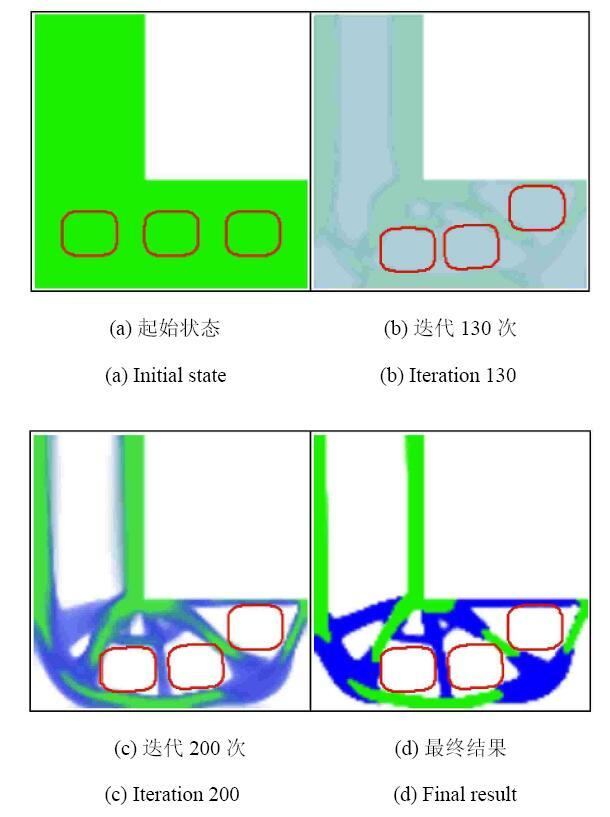Fig. 9   Optimization results of L-shaped beam with three embedded holes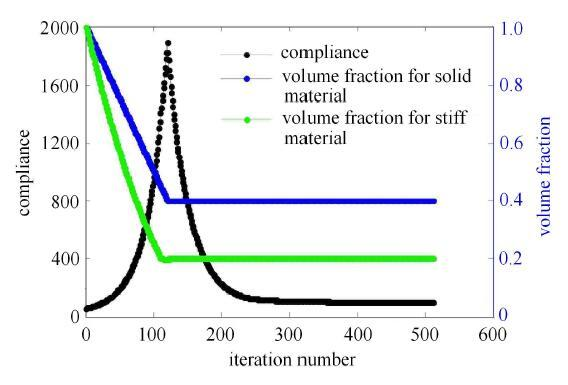Fig. 10   Convergence histories of the objective function and volume fraction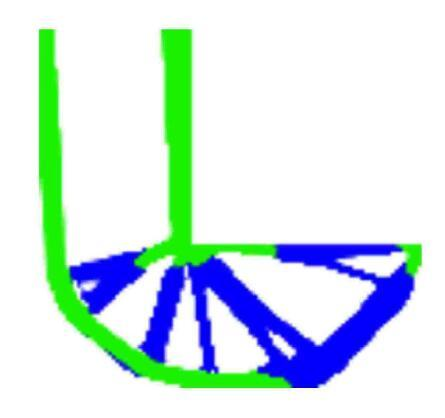Fig. 11   Optimization results of L-shaped beam without embedded holes

$E_e = \left( {\rho _1^e } \right)^{p_1 }\Big \{ \left( {\rho _2^e } \right)^{p_2 }\Big[ \left( {\rho _3^e } \right)^{p_3 }E_3 + \left( {1 - \left( {\rho _3^e } \right)^{p_3 }} \right)E_2 \Big ]+$

$\qquad \left( {1 - \left( {\rho _2^e } \right)^{p_2 }} \right)E_1 \Big \} \eta _e^q (21)$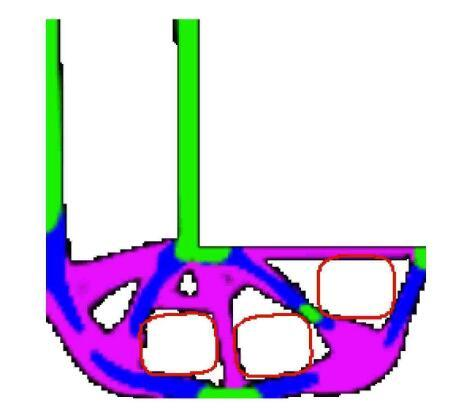Fig. 12   Optimization results of L-shaped beam with three embedded holes

## 5 结 论

The authors have declared that no competing interests exist.

## 参考文献 原文顺序 文献年度倒序 文中引用次数倒序 被引期刊影响因子

(Zhang Weihong, Guo Wenjie, Zhu Jihong.Integrated layout and topology optimization design of multi-component systems with assembly units. Acta Aeronauticaet Astronautica Sinica, 2015, 36(8): 2662-2669 (in Chinese))〈〉